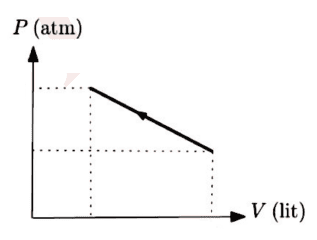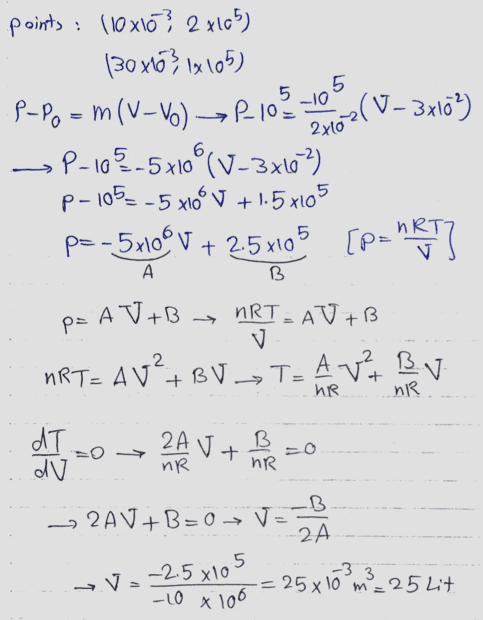# Question's Diagram: Am I Right?

• MatinSAR

#### MatinSAR

Homework Statement
We condense some ideal gas from a volume of 30 liters and a pressure of 1 atmosphere to a volume of 10 liters and a pressure of 2 atmospheres. What is the volume of the gas when its temperature is maximum?
Relevant Equations
pV=nRT
Hello. This is question's diagram.Can someone tell me if I am wrong ?Looks ok, except that you did not check that it is a maximum. Could be a local minimum, in which case either the starting or finishing temperature would be the max.

•MatinSAR
Homework Statement:: We condense some ideal gas
Presumably you mean 'compress'. 'Condense' means something different!

It's not necessary to use SI units here. You can work in 'atm' and 'lit' (though 'lit' is not a common abbreviation for litres) and you will get the same answer. It's less messy.

For example, the gradient of the line can immediately be written as ##\frac {-1}{20}## (corresponding to units of atm/lit).

Try it – and check you understand why it works here.

•MatinSAR
Looks ok, except that you did not check that it is a maximum. Could be a local minimum, in which case either the starting or finishing temperature would be the max.
I will use derivative test to prove it.
•